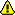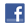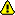New account
4 million accounts created!

• Home
• Report a bug

•

Learn German > German lessons and exercises > German test #1957> Other German exercises on the same topic: Numbers [Change theme] > Similar tests: - Numbers: 0-100 - Counting from 1 to 10 - Numbers from 20 to 100 - Numbers from 11 to 20 - Numbers - Counting from 100 to 1 000 - 100-100,000,000,000 - Maths > Double-click on words you don't understand

# Maths

Maths

+ plus

- minus

* mal

durch (you can also use: dividiert durch)

Result: 'ist' OR (occasionaly)'ergibt' or 'macht'

Examples

Four plus two equals six = Vier plus zwei ist sechs.

Ten minus five equals five = Zehn minus fünf ist fünf.

Three times three equals nine = Drei mal drei ist neun.

Five divided by five equals one = Fünf durch fünf ist eins.

Exercise

Write down the full sentence in German.Please use SEIN .Twitter Share
German exercise "Maths" created by micka with The test builder. [More lessons & exercises from micka]
Click here to see the current stats of this German testPlease log in to save your progress.

To insert special letters:

Five minus two equals three =» .

Seven plus nine equals sixteen =»

Seven times twelve equals eighty-four =»

Twenty-five divided by five equals five =»
.

Twenty-two minus thirteen equals nine =» .

Thirty-four plus forty-one equals seventy-five. =»

Twenty divided by ten equals two.

Null times twelve equals null =»

Seventeen plus eight equals twenty-five. =»

Forty-six minus twenty-eight equals eighteen. =»

End of the free exercise to learn German: Maths
A free German exercise to learn German.
Other German exercises on the same topic : Numbers | All our lessons and exercises